# Programming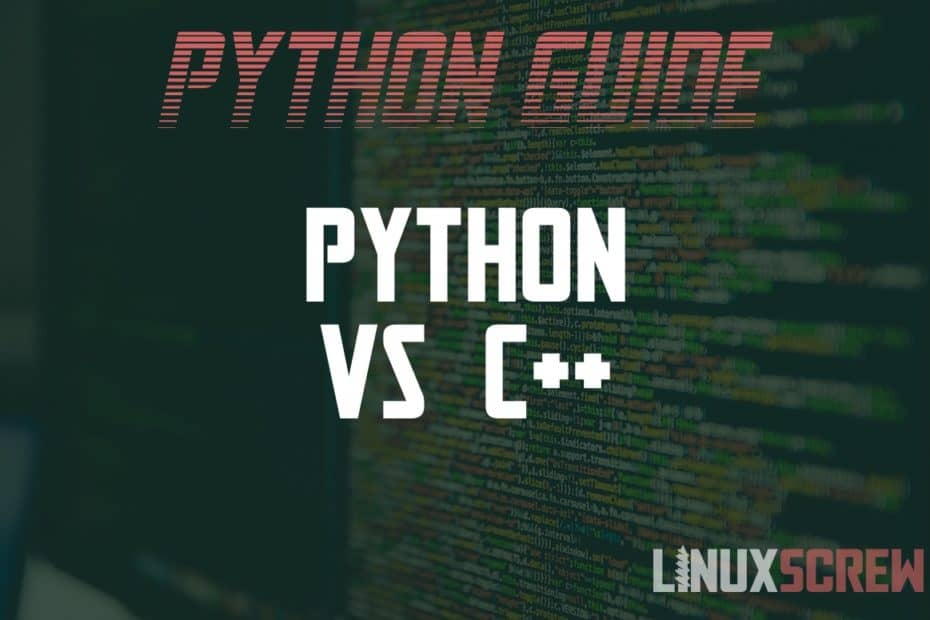## C++ vs Python – Which Programming Language to Learn in 2022?

• Python

Here’s a quick primer on the C++ and Python Programming languages to help the beginner or intermediate coders decide which to learn. What is C++? C++ is a compiled general-purpose programming language. It’s an extension of the C programming language – using similar syntax but adding object-oriented programming features like classes,… Read More »C++ vs Python – Which Programming Language to Learn in 2022?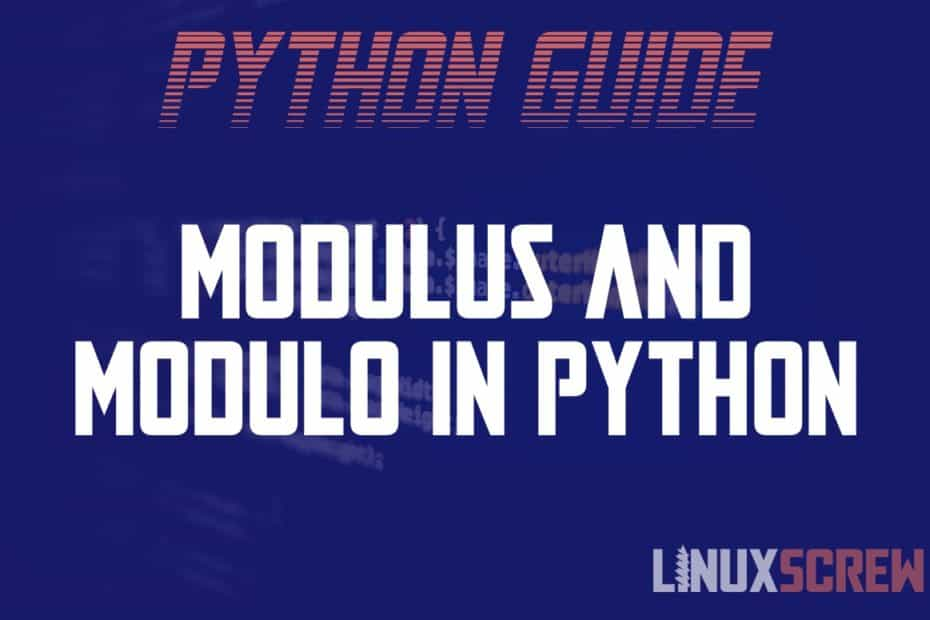## Modulus (Mod), Modulo and Python – Explanation and Code Examples

• Python

This tutorial will explain (hopefully in the simplest terms possible) what modulus and modulo mean and how they can be calculated in the Python programming language. Modulus and Modulo are Different Things! There’s a bit of confusion about this, and it seems that in some places, the terms… Read More »Modulus (Mod), Modulo and Python – Explanation and Code Examples## Modulus (Mod), Modulo and PHP – Explanation and Code Examples

• PHP

This article will explain (in the simplest terms possible) what modulus and modulo mean and how they are calculated in the PHP programming language. Modulus and Modulo are NOT the Same Things! There’s a bit of confusion about this, and it seems that in some places, the terms… Read More »Modulus (Mod), Modulo and PHP – Explanation and Code Examples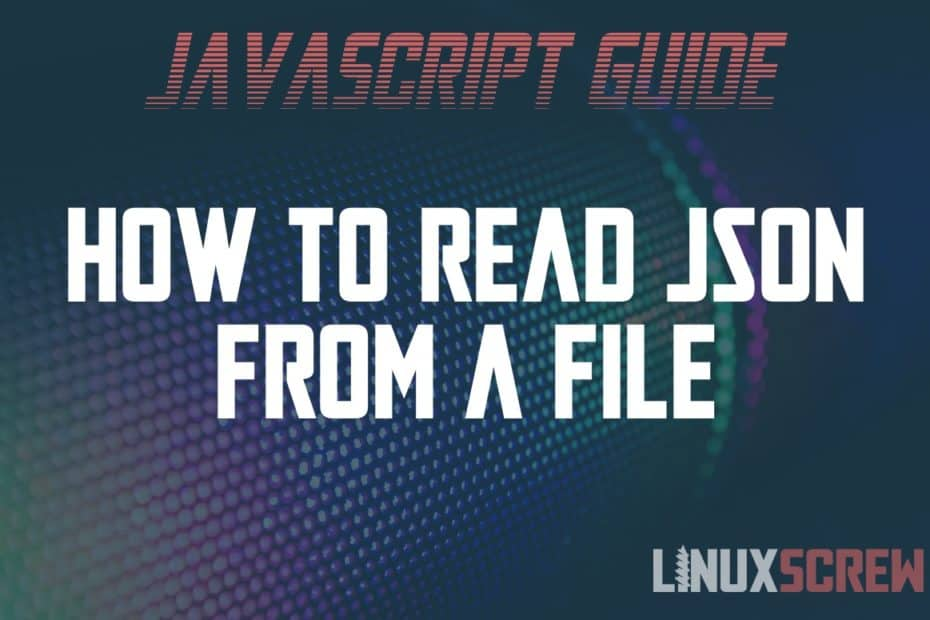## How to Read a Local/Remote JSON File in JavaScript [Examples]

• Javascript

This article will show you how to read a JSON file into JavaScript as a JSON object – both local and remote files. What is JSON? JSON (JavaScript Object Notation) is a file format that stores objects, and arrays of objects, as human-readable text. It’s become the… Read More »How to Read a Local/Remote JSON File in JavaScript [Examples]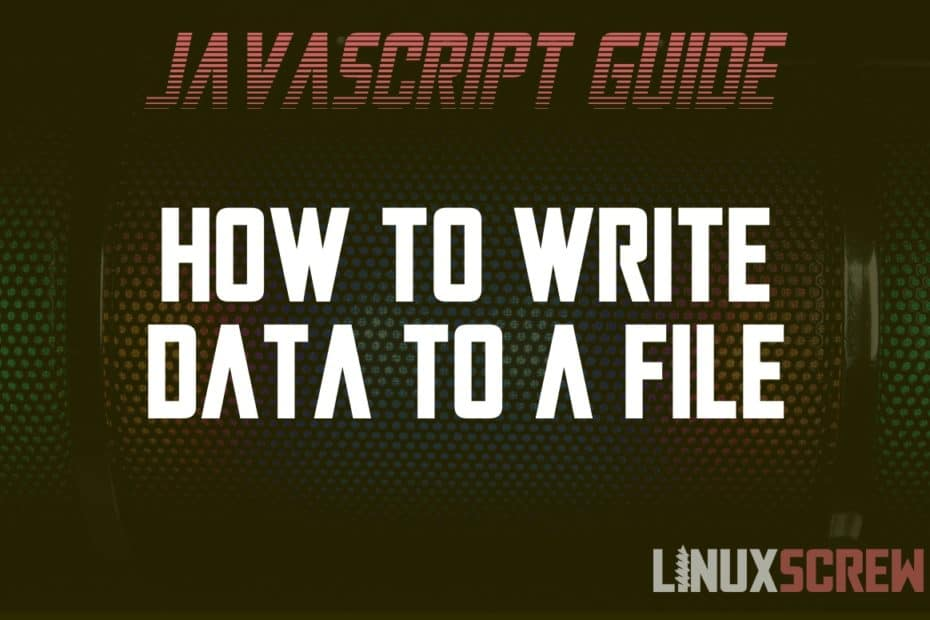## How to Write to a File Using JavaScript, With Examples

• Javascript

This article will show you how to write to files from JavaScript – both from the web browser and Node.js environments. With examples on how to write, append, update, delete and rename files. JavaScript code is usually run from one of two environments – within a… Read More »How to Write to a File Using JavaScript, With Examples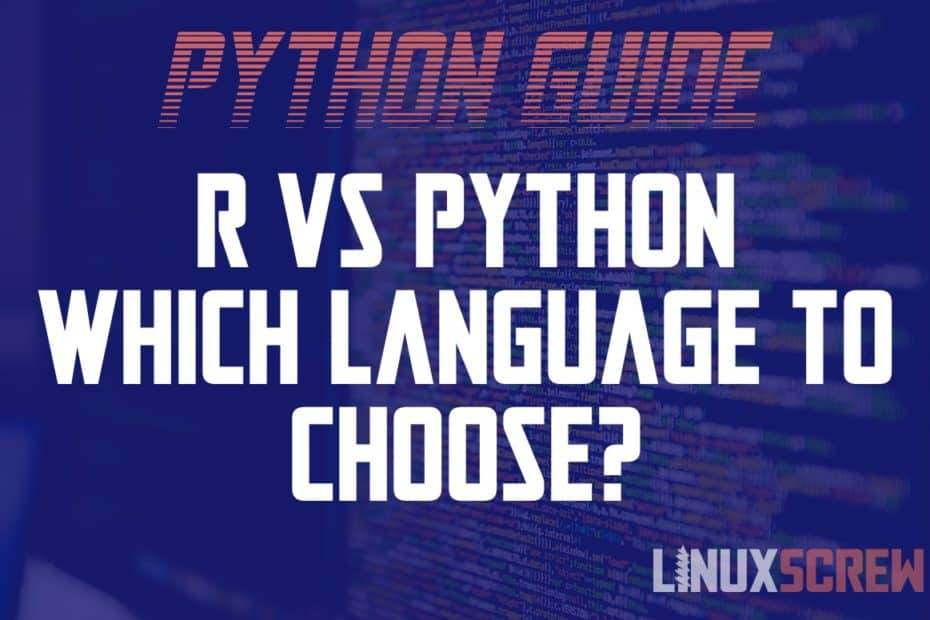## R vs Python – What Makes them Different? Which is Best?

• Python

This article provides a simple comparison of the R and Python programming languages – and what they’re best used for. If you’re looking to learn to program and are interested in data analysis, you’ll probably run into the choice – R or Python? This article breaks down each and why you might… Read More »R vs Python – What Makes them Different? Which is Best?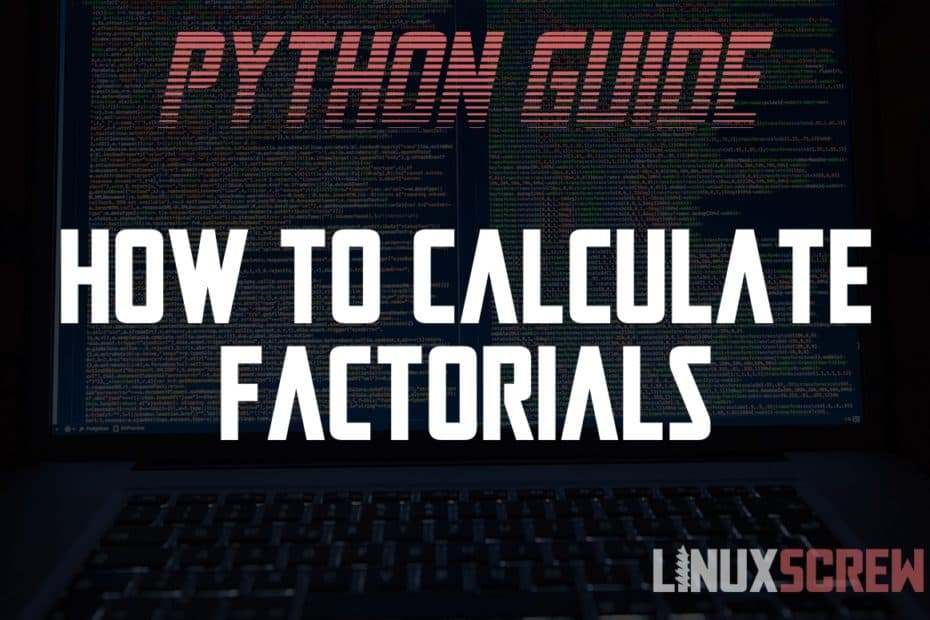## Calculating Factorials in Python – The Easy Way

• Python

This short article will show you the quickest and easiest way to calculate a factorial in Python using the factorial() function. This article is written for the Python 3 programming language. What is a Factorial, and How is it Calculated? A factorial is a mathematical formula in which a given positive integer is… Read More »Calculating Factorials in Python – The Easy Way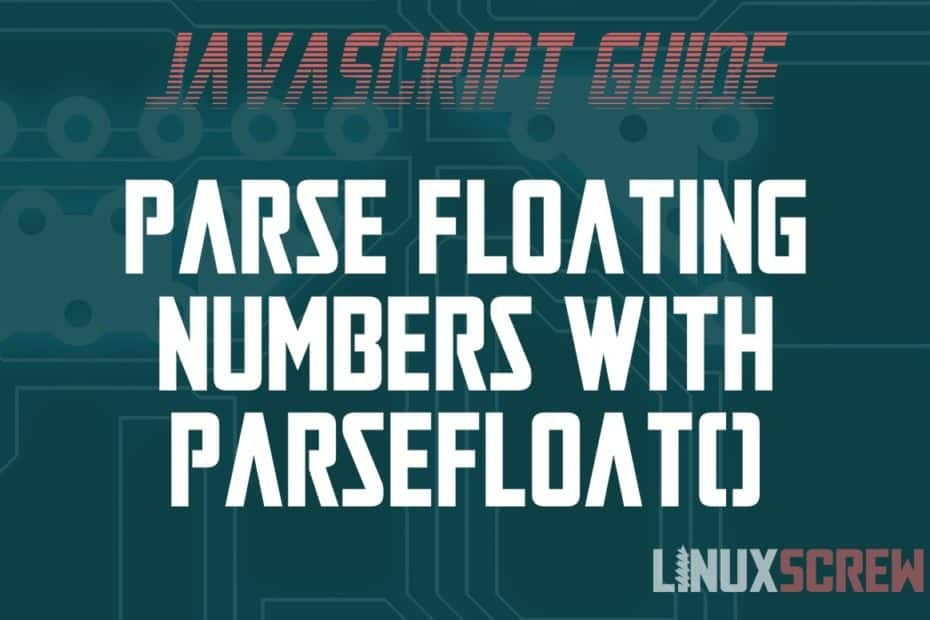## Converting to Float Numbers with the parseFloat() JavaScript Function, With Examples

• Javascript

This article will explain floating-point numbers and how to convert values to floating-point numbers using parseFloat() in JavaScript. Looking to convert to an integer value instead – use parseInt()! What is a Floating Point Number? In programming, a floating-point number (commonly just called a float) is a number with any number of characters… Read More »Converting to Float Numbers with the parseFloat() JavaScript Function, With Examples## How to Compare Dates in PHP, With Examples

• PHP